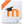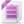Topic outline

•Algebra 1 (B)

The main goal of Algebra is to develop fluency in working with linear equations. In this course, students will work with tables, graphs, and equations and solve linear equations and inequalities and systems of linear equations and inequalities. Students will learn how to simplify polynomials and begin to study quadratic relationships, along with analyzing mathematical situations verbally, numerically, graphically, and symbolically. Throughout the course, students will have opportunities to apply mathematical skills and make meaningful connections to life’s experiences.•Algebra 1B Moodle Course File
•Algebra 1B Common Cartridge 1.1 Course File
•Week 1

•LEARN: Power of Powers URL
•Week 2

•PRINT: Checklist for Week 2 URL
•WATCH: Multiplication of Monomials Page
•WATCH: Division of Monomials Page
•Week 3

•WATCH: Scientific Notation Page
•WATCH: Operations with Scientific Notation Page
•LEARN: Introduction to Polynomials URL
•LEARN: Multiplying by a Monomial URL
•Week 4

•PRINT: Checklist for Week 4 URL
•LEARN: Multiplying by a Polynomial URL
•LEARN: Multiplication Shortcuts URL
•WATCH: Multiplication Shortcuts Page
•Week 5

•LEARN: Factors URL
•WATCH: Factoring Monomials & Finding GCF of Polynomials Page
•LEARN: Using the Distributive Property URL
•Week 6

•INTRODUCTION to Week 6 Page
•WATCH: Factoring Trinomials II Page
•WATCH: Practice Factoring Trinomials Page
•Week 7

•INTRODUCTION to Week 7 Page
•PRINT: Checklist for Week 7 URL
•Week 8

•WATCH: Maximum and Minimum Values URL
•WATCH: Example of Graphing Quadratic Equations Page
•WATCH: Vertical and Horizontal Shifts of Quadratic Equations Page
•WATCH: Dialations of Quadratic Graphs Page
•WATCH: Solving Quadratic Equations by Graphing (1 Root) URL
•Week 9

•PRINT: Checklist for Week 9 URL
•LEARN: Solving Quadratic Equations URL
•WATCH: Solving Quadratic Equations Page
•Week 10

•WATCH: Practice Solving Quadratic Equations by Factoring Page
•WATCH: Additional Examples Solving Quadratic Equations by Factoring Page
•WATCH: Review Solving Quadratics- 2 Ways Page
•WATCH: Solving Quadratics not in Standard Form Page
•Week 11

•Week 12

•INTRODUCTION to Week 12 Page
•PRINT: Checklist for Week 12 URL
•OPTIONAL VIDEO: Additional Examples of Operations with Rational Expressions Page
•LEARN: Dividing a Polynomial by a Monomial URL
•Week 13

•Week 14

•LEARN: Multiplying Radical Expressions URL
•WATCH: Solving Radical Equations Page
•Week 15

•LEARN: Distance Formula URL
•WATCH: Unit 8 Review- Radical Expressions & Equations Page
•CRT Review

•Week 17

•